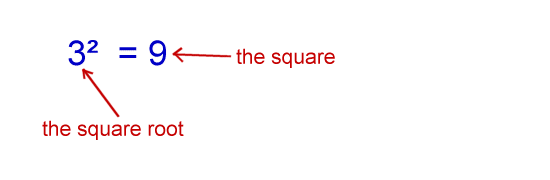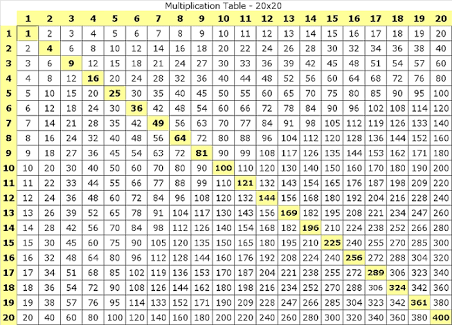# Square and Square Root One or Two Digits (Primary 4)

MATHEMATICS

SECOND TERM

WEEK 2

PRIMARY 4

THEME – BASIC OPERATIONS

PREVIOUS LESSON – Multiplication of Whole Numbers by 2 Digit Number not Exceeding 50 (Primary 4)

TOPIC – SQUARE AND SQUARE ROOT ONE OR MORE

LEARNING AREA

1. Square One or Two Digits

2. Square Root of Perfect Squares up to 400

3. Quantitative Reasonings

PERFORMANCE OBJECTIVES

By the end of the lesson, the pupils should have attained the following objectives (cognitive, affective and psychomotor) and should be able to –

1. calculate the squares of 1 and 2 digit numbers.

2. solve quantitative aptitude problems involving squares.

3. find square roots of perfect squares up to 400.

4. solve quantitative aptitude problems of square roots of perfect squares not greater than 400.

ENTRY BEHAVIOUR

Multiplication of Numbers

INSTRUCTIONAL MATERIALS

The teacher will teach the lesson with the aid of:

1. 20 by 20 square chart

2. Charts on quantitative reasoning.

3. Square root chart.

METHOD OF TEACHING – Choose a suitable and appropriate methods for the lessons.

Note – Irrespective of choosing methods of teaching, always introduce an activities that will arouse pupil’s interest or lead them to the lessons.

REFERENCE MATERIALS

1. Scheme of Work

2. 9 – Years Basic Education Curriculum

3. Course Book

4. All Relevant Material

5. Online Information

CONTENT OF THE LESSON

## LESSON 1 – INTRODUCTION

Pupil’s Activities 1 – Copy and Complete the table.Teacher’s remark – A number multiple by itself is called a square number. Square numbers are formed by multiplying a whole number by itself. 1 x 2 = 1, 2 x 2 = 4, 3 x 3 = 9, 4 x 4 = 16, 5 x 5 = 25, etc. are square numbers. A number squared means a number multiplied by itself.

Teacher’s/Pupil’s Activities 2 – Writing and Pronunciation of Square of Numbers

2 x 2 is written as 2² and pronounced as 2 square. Let the pupils repeat after you as you pronounce 2².

4 x 4, as 4² and pronounced as 4 Square.

Pupil’s Activities 3 – Write 1 to 20 as square and Pronounce

Teacher activities – Pick the pupils randomly to pronounce the square of numbers.

Teacher’s/Pupil’s Activities 4 – Guide the pupils to find the square of the following numbers.

(1) 11

(2) 23Pupil’s Activities 5 – Class Exercises/Assignments

Find the square of the following numbers –

1. 9

2. 45

3. 72

## LESSON 2 – QUANTITATIVE REASONING ON SQUARE OF NUMBERS

As stated in their quantitative reasoning or textbooks.

## LESSON 3 – SQUARE ROOT OF PERFECT SQUARES UP TO 400

Pupil’s Activities 1 – Study the following numbers and discuss your observations.

1. √4 = √(2 x 2) = 2

2. √9 = √(3 x 3) = 3

3. √16 = √(4 x 4) = 4

Teacher’s remark – This sign (√)  is called square root sign. Square root of 4 is written as √4. The square root is the opposite of the square. Square root of a number is the “root” of the square. That’s, the number that was used to make the square.Teacher’s/Pupil’s Activities 2 – Guide the pupils to find the square root of Numbers

Find the Square Root of the following numbers –

1. 25

2. 121Pupil’s Activities – Class Exercises/Assignments

Find the square roots of the following numbers –

1. 100

2. 144

3. 225

## LESSON 4 – QUANTITATIVE REASONING ON SQUARE ROOTS

As stated in their quantitative books or Textbooks.

PRESENTATION

To deliver the lesson, the teacher adopts the following steps:

1. To introduce the lesson, the teacher revises the previous lesson. Based on this, he/she asks the pupils some questions;

2. Guides pupils to find the square of a number say 3 by locating the intersection of 3 by 3 on the vertical and horizontal rows and columns respectively on the square chart to get 9.

Pupil’s Activities – Use square chart to find squares of any given number.

3. Guides pupils to solve quantitative aptitude problems involving square of 1-digit and 2-digit numbers.

Pupil’s Activities – Solve series of quantitative aptitude problems involving squares of 1-digit and 2-digit numbers.

3. Guides pupils in locating the square root of any perfect square on the chart e.g. square root of 256 is 16.

Pupil’s Activities – Find square root of perfect squares not exceeding 400 using the square root chart.

4. Guides pupils to solve quantitative aptitude problems involving square root of perfect squares not greater than 400, illustrating with charts.

Pupil’s Activities – Solve quantitative aptitude involving square root of numbers not exceeding 400.

5. Class exercises/Assignments

CONCLUSION

• To conclude the lesson for the week, the teacher revises the entire lesson and links it to the following week’s lesson.
• Next Lesson –

LESSON EVALUATION

Pupils to:

1. find the square of given number using 20 by 20 square chart.

2. solve quantitative aptitude problem involving finding of squares.

3. find square root of given numbers by using 20 by 20 square chart.

4. find square root of perfect squares using factor method.

5. solve quantitative aptitude problems involving squares and square root of numbers.